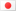Understanding Machine Learning…Nithin K February 20, 2018日本語Machine Learning, Artificial intelligence, Data Analytics, Deep Learning …. Have you been hearing many jargons like these, more strongly than ever these days, yet have no clear idea what they are all about? Well, this blog is exactly for you!

Artificial intelligence is all about introducing intelligence to a computer by providing historical data and predicting the future based on the same. For example, whenever a computer encounters a cat’s image, it will understand that the image is that of a cat by comparing it with a set of images of animals in its database and by arriving at a category name which is having the highest probability match, which is cat here.

Artificial intelligence is a broad term which has many branches – Machine Learning, Deep Learning, Data Analytics, Expert systems and beyond. All these are basically the sciences of programming computers to learn by themselves based on the data provided and predicting the results/performing the actions accordingly. Yet, there are slight differences in each, mainly with respect to the applications which employ each of these technologies.You might have heard about the Google car project running for the last couple of years. Why is the reason behind the long duration? It is actually collecting the data for possible instances a car/driver may face and based on these captured instances, intelligence is imparted artificially to the car, to enable it to drive on its own, without human intervention. Some of the other applications of AI are Speech Recognition, Face Recognition, Predicting web search results, Recommending videos based on past search history, Beating world champions in various games like Go/Chess and more.

## Traditional Computer Programming vs Machine Learning

IBM’s Deep Blue beat world champion Garry Kasparov in 1997. Did Deep Blue use Machine Learning? Hmmm…. No! Deep Blue Super Computer didn’t learn anything while playing with Kasparov. The logic was pre-programmed by human programmers. Then what is Machine Learning!!!

The base of Machine Learning is preconceived data. It will always have a Training phase and a Testing phase. Intelligence is created in the training phase. The Google car project was running for several years as part of training. ImageNet project collected millions of images from the internet and acted as a DB for training image classification models for animals, birds, buildings and various other objects in the world. The predictions based on the trained model happen in the testing phase. Two kinds of predictions occur in general:

Regression is used to predict continuous values.

•  Given a set of data, find the best relationship that represents the set of data.
•  Forecast a value

Classification is used to predict which class a data point is part of (discrete value).

## Linear Regression Algorithm

Let’s try to understand a simple regression model “Linear Regression” with an example. We plotted the incomes of a set of people on the basis of their education as shown below.You can see a general increasing trend of income with respect to their education. And there is a bias “b”; even for an uneducated person, there will be a minimum income right? The objective of linear regression algorithm is to draw the optimum trend line so that whenever a new person with known educational details turns up, we would be able to predict his income with certain level of confidence.

Let’s leap into the internals of algorithm. We can plot the line with the equation Y = aX+b+U, where “Y” is Income (dependent variable) and “X” is Education (independent variable). “A” is the slope or the rate of increase of income with respect to education and “U” is the total sum of error. What does error mean here? When the algorithm tries to plot a line, some points (or a large number of points) may not touch the line plotted. The difference between the line and the points is the error or otherwise the prediction is far from reality by this much difference. “U” is the total sum (or better accurate methods like RMSE) of these differences. So the objective of the algorithm is to find the value of “a” and “b” when U is at its minimum. Linear regression tries random values for “a” and “b” and observes the value of U. Whenever it reaches a minimum value, it stops experimenting further values and takes the values of a and b and creates the equation aX+b (ex: 5X+3). Prediction is now over. If we know education (X), we will get the income of that person. In reality, it is not just one independent variable but many (a1x1 + a2x2 + a3x3 + b).

When you have good understanding of simple models like the above, more complex classification models like neural networks welcome you to dive into the world of AI (which is used more in deep learning areas such as image recognition, face recognition, speech recognition etc.) with a quote “Unless there is no historical data, there is no machine learning…”